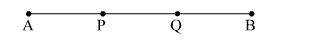# Find the coordinates of the points of trisection of the line segment joining the points A(7, –2) and B(1, –5).

Question:

Find the coordinates of the points of trisection of the line segment joining the points A(7, –2) and B(1, –5).

Solution:Consider the figure.
Here points P and Q trisect AB.
Therefore, P divides AB into 1 : 2 and Q divides AB into 2 : 1.
Using section formula, coordinates of P are;

$\mathrm{P}(x, y)=\left(\frac{1 \times 1+2 \times 7}{3}, \frac{1 \times-5+2 \times-2}{3}\right)$

$\mathrm{P}(x, y)=\left(\frac{15}{3}, \frac{-9}{3}\right)=(5,-3)$

Similarly, coordinates of Q are;

$\mathrm{Q}(a, b)=\left(\frac{2 \times 1+1 \times 7}{3}, \frac{2 \times-5+1 \times-2}{3}\right)$

$\mathrm{Q}(a, b)=\left(\frac{9}{3}, \frac{-12}{3}\right)=(3,-4)$

Therefore, coordinates of points P and Q are (5, -">-3) and (3, -">-4) respectively.